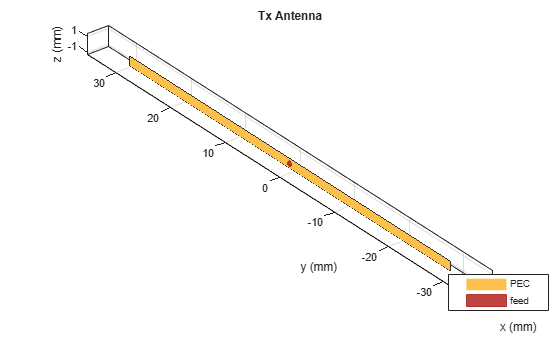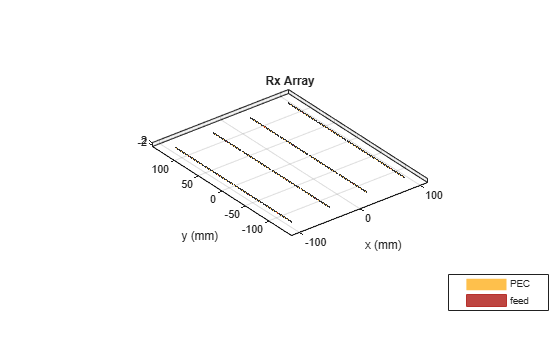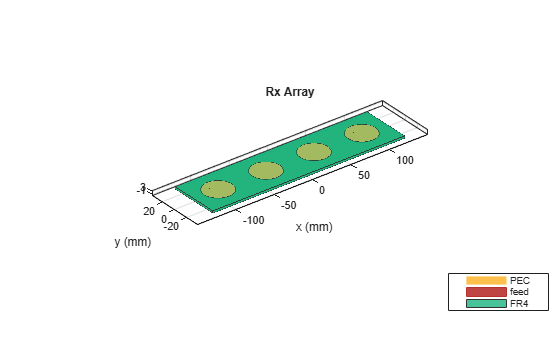# Direction of Arrival Determination Using Full-Wave Electromagnetic Analysis

This example shows how to determine the Direction of Arrival (DoA) when the transmission source is an antenna located in the far-field region or by assuming that the incident signal behaves like a plane wave incident on the receive array. DoA can be denoted by two angles phi and theta in the spherical co-ordinate system. It is a common practice to use a receive antenna array to scan for any incoming signals and to calculate its angles of arrival with respect to the center of the array. The incoming signal is commonly assumed to arrive from a far-field transmission source. Proper accounting of receive array’s electromagnetic behavior is one of the key elements in determining the angles of arrival of the incident signal.

The receiving array for DoA determination can either be a linear or a rectangular array. As the transmit antenna lies in the far-field with respect to the receive array, the mutual interaction between the transmit antenna and the receive array can be neglected. However, there is a finite amount of mutual coupling between the respective elements of the receive array. Mutual coupling is one of the key elements that impact the DoA determination. Mutual coupling depends on various factors such as the type of antenna elements selected used in the receive array, the orientation and separation of the respective array elements, along with the material properties (like conduction loss and dielectric loss) of the antenna elements. For determining the operational boundaries of the the DoA system, it is essential to consider the configuration of the receive array first. You can use the `doa` function from Antenna Toolbox™ to perform full-wave electromagnetic analysis and calculate angles of arrival for a given receive array configuration and type of antenna elements. This aids the designers in selecting the optimal receive array configuration and the optimal antenna elements for a DoA system design. The `doa` function consists of two input arguments, the first argument is either a `conformalArray` object or a `planeWaveExcitation` object, while the second argument is the operating frequency of the system (either a single frequency value or vector of values). The output argument of `doa` provides the calculated phi and theta angles in spherical co-ordinates.

### DoA Computation for Conformal Array

This example shows a use case where the input argument is a `conformalArray` object. The `conformalArray` object consists of two elements. The first element is a single feed transmit antenna lying in the far-field with respect to the receive array. The second element is a homogenous uniformly spaced linear or rectangular receive array, with an even number of antenna elements. In this example, the receive array is implemented as a rectangular array.

#### Assign Operational Frequency

Set the desired frequency (F0) to 2.4 GHz and compute the corresponding wavelength (lambda) using the speed of light and frequency.

```F0 = 2.4e9; lambda=physconst('LightSpeed')/F0;```

#### Create Transmit Antenna Center Offset Location

Set the location of the transmit antenna in the far-field region by providing arbitrary azimuth and elevation angle values of ${0}^{\circ }$ and ${30}^{\circ }$, respectively.

```r=100*lambda;% far-field region az=0; el=30; tx=r*cosd(az)*sind(el); ty=r*sind(az)*sind(el); tz=r*cosd(el); % Offset of Tx antenna center from the origin Tx_center_offset=[tx,ty,tz]; ```

#### Create and Visualize Transmit Antenna Geometry

Create the geometry of the single feed transmit antenna element and visualize it using the `show` function. In this example, a dipole antenna element is used and placed along the Z-axis. To set the orientation of the dipole antenna as horizontal, set the parameter `Tilt` to ${90}^{\circ }.$ Generate the physical layout of the dipole antenna using the design function from Antenna Toolbox™.

```ant1=design(dipole,F0); ant1.Tilt=90; %To make it horizontal figure; show(ant1); title('Tx antenna')```#### Create Receive Array Center Offset Location

The offset location of the receive array is assumed at the origin of the co-ordinate system.

`Rx_center_offset=[0,0,0]; `

#### Create and Visualize 4 by 4 Rectangular Receive Array Geometry

The homogenous uniform receive array is constructed using the catalog element `rectangularArray` from Antenna Toolbox™. The rectangular array consists of dipole antenna elements. Set the size of the array to 4 by 4. Specify even number of elements along each axis of the array. Set the spacing between the two adjacent elements to lambda/2 along each axis. Visualize the receive array using the `show` Function.

```RxArray2=rectangularArray('Size',[4 4]); RxArray2.Element = design(dipole,F0); RxArray2.RowSpacing=0.5*lambda; RxArray2.ColumnSpacing=0.5*lambda; ant2=RxArray2; ant2.Element.Tilt=90; %To make it horizontal figure; show(ant2) title('Rx array')```#### Create Conformal Array Geometry

Create a `conformalArray` object and assign the transmit and receive elements to it. The transmit element is the first element and the receive element is the second element. Here, the transmit element is a single dipole antenna and the receive element is a uniform homogenous 4 by 4 rectangular array of dipole antennas.

```c=conformalArray; c.ElementPosition=[Tx_center_offset;Rx_center_offset]; %1st element of conformal array is Tx %2nd element of conformal array is Rx c.Element={ant1 ant2};```

#### Call `doa` for Conformal Array Object

Call the `doa` function on the `conformalArray` object.

`[phiArrival1, thetaArrival1]= doa(c, F0) `
```phiArrival1 = -2.9205e-05 ```
```thetaArrival1 = 29.1207 ```

The results show a slight difference in the calculated angles `phiArrival1` and `thetaArrival1` than the previously specified location of the transmitter. You can do this analysis by using alternate receive array configurations and antenna elements to select the optimum configuration that fits the accuracy requirements for your DoA system.

### DoA Computation for Incident Plane Wave

This example shows the DoA computation for an incident plane wave signal.

#### Create Single Element of Homogenous Receive Array

Create a circular microstrip patch antenna operating at 2.4 GHz as the receive array element by using the `design` function from Antenna Toolbox™. Assign the ground plane dimensions to it. Add a lossy FR4 dielectric substrate to it. Adjust the thickness of the dielectric substrate and the radius of the circular microstrip patch to achieve a resonant frequency of 2.4 GHz.

```RxElement = design(patchMicrostripCircular, F0); RxElement.GroundPlaneLength = lambda/2; % in m RxElement.GroundPlaneWidth = lambda/2; % in m RxElement.Radius = 0.018;% in m prx_con.Height = 0.0016;% in m RxElement.FeedOffset = [ -0.006 0.006];% in m RxElement.Substrate = dielectric('FR4'); % lossy substrate```

#### Create and Visualize Linear Array as Receive Array

Construct a homogenous uniform receive array using the `linearArray` element in the array catalog. Set the total number of array elements to 4. Set the spacing between adjacent antenna elements to `lambda/2.` View the array geometry using the `show` function.

```RxArray1 = linearArray; RxArray1.Element = RxElement; RxArray1.NumElements = 4; RxArray1.ElementSpacing = lambda/2; ant2=RxArray1; figure; show(ant2) title('Rx array')```#### Create Direction and Polarization Vectors for Plane Wave Excitation

Set the position angles of the incident plane wave to ${10}^{\circ }$ azimuth and ${30}^{\circ }$ elevation. Using these angles, determine the direction and polarization vectors of the incident plane wave.

```az_ref=10; el_ref=30; dir=[sind(el_ref)*cosd(az_ref) sind(el_ref)*sind(az_ref) -cosd(el_ref)]; pol=[cosd(el_ref)*cosd(az_ref) cosd(el_ref)*sind(az_ref) sind(el_ref)];```

#### Create the `planeWaveExcitation` Object

Create the `planeWaveExcitation` Object with the previously created linear array as its receive element and direction and polarization vectors as calculated above.

```obj1 = planeWaveExcitation('Element', ant2, 'Direction', ... dir,'Polarization', pol);```

#### Call `doa` for the planeWaveExcitation Object

Call the `doa` function on the `planeWaveExcitation` object to compute the angles of arrival of the incident plane wave.

`[phiArrival2, thetaArrival2]= doa(obj1, F0)`
```phiArrival2 = 10 ```
```thetaArrival2 = 31.2847 ```

Like the computation in the previous example, the computed `phiArrival2` and `thetaArrival2 `values in this example slightly differ from the specified azimuth and elevation angles. These values can be used to study the trade-offs between different array elements and configurations and to select the optimum configuration for your use case. This analysis can be used either for calibrating the DoA system or as an input to signal processing-based DoA algorithms. The finite difference between the actual and predicted angles depends on the electromagnetic properties of the receiving array. Antenna Toolbox™ uses full-wave electromagnetic solution of Maxwell's equations to model the behavior of antenna systems and as shown, can be used for predicting direction of arrival using the `doa` function.

### Conclusion

With Antenna Toolbox™, you can perform a similar analysis with antenna elements in the antenna catalog or custom antenna elements constructed from the shape primitives, `pcbstack` or imported via the `stl` function. This workflow facilitates the study of DoA system configurations from strictly a full-wave electromagnetic analysis viewpoint. This analysis is useful to initially assess the types of antenna elements and antenna array configuration that are used to achieve a desired overall system performance for a DoA system.